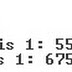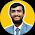# C/ C++ Programming Practice Problem Question with Solution Loop If else

Loop If else condition C/ C++ Programming Practice Problem Question with Solution

1. Write a program in c++ that swap two number without using any extra variable.   An example running session looks as follow:
Solution:
#include <iostream>
using namespace std;
int main() {
int num1,num2;
cout << "Enter Number 1 :";
cin >> num1;
cout << "Enter Number 2 :";
cin >> num2;
cout << "Before Swap Number is 1: "<<num1<<" & Number 2 is :"<<num2<<endl;
num2=num1+num2;
num1=num2-num1;
num2=num2-num1;
cout << "After Swap Number is 1: "<<num1<<" & Number 2 is :"<<num2;
cin.get();
return 0;
}

# 2.Write a program that input for students’ final grades in a given class (the grades are integer values in the range 1-9) after each time the program prompts "Do you want enter more Grade Y/N :" until user press N. The program then displays the class grade average, the highest grade, and how many students in the class failed the course (a grade less than 4 is a failing grade).. An example running session looks as follow:

Solution:
#include <iostream>
using namespace std;
int main() {
int grade, count, highest, failed;
double sum, average; count = 0;
char choice;
sum = 0;
highest = 0;
failed = 0;
do{
cout << "Enter student's grade:";
cin >> grade;
if( grade >=1 && grade <=9 )
{
sum = sum + grade;
if ( grade > highest )
highest = grade;
if ( grade < 4 )
++failed;
++count;
}
else
cout << "Invalid grade Input\n";
cout << "Do you want enter more Grade Y/N :";
cin >> choice;
}while ( choice=='Y'||choice=='y' );
average = sum / count; cout << endl;
cout << "Class average: " << average << endl;
cout << "Highest grade: " << highest << endl;
cout << "Number of students that failed the course: " << failed << endl;
cin.get();
return 0;
}

`3. Write a program in c++ that prints a 'pyramid' shape of a specified height on the screen. Your height must be between 1 and 30`
` `

Solution:

#include <iostream>
using namespace std;
int main()
{
int pyramid_height;

cout << "How high would you like the pyramid?: ";
cin >> pyramid_height;
while (pyramid_height > 30 || pyramid_height < 1)
{
cout << "Pick another height (must be between 1 and 30): ";
cin >> pyramid_height;
}

int line;
cout <<endl;
for (line = 1 ; line <= pyramid_height ; line++)
{
int count;
int total_no_of_spaces =  pyramid_height - line;

for (count = 1 ; count <= total_no_of_spaces ; count++)
cout << ' ';

for (count = 1 ; count < line * 2 ; count++)
cout << '*';

cout <<endl;
}
cin.get();
return 0;
}

4. Write a program in c++  that read a number from user print its reverse number.

Solution:

#include <iostream>
using namespace std;
int main()
{
int n,sum=0,rem;
cout<<"Enter No:";
cin>>n;
cout<<"Orginal No:"<<n;
while(n>0)
{
rem=n%10;
sum=sum*10+rem;
n /= 10;
}

cout<<"\nRevesed No:"<<sum;
cin.get();
return 0;
}

5. Write a program that print the following menu and get a user choice and execute the program.

*****Menu****

1.Swap Number
2.Grade
3.Pyramid
4.Reverse
Enter a User choice:

Hint: Use switch statement or for exit from program use “exit (1);” statement.

Solution:
#include <iostream>
using namespace std;
int main()
{
int choice;

do
{
cout<<"\n*****Menu****\n";
cout<<"1.Swap Number\n";
cout<<"2.Grade \n";
cout<<"3.Pyramid\n";
cout<<"4.Reverse\n";
cout<<"5.Exit\n";
cout<<"Enter a choice: ";
cin>>choice;
switch(choice)
{
case 1:
cout << "\n*******************************\n";
int num1,num2;
cout << "Enter Number 1 :";
cin >> num1;
cout << "Enter Number 2 :";
cin >> num2;
cout << "Before Swap Number is 1: "<<num1<<" & Number 2 is :"<<num2<<endl;
num2=num1+num2;
num1=num2-num1;
num2=num2-num1;
cout << "After Swap Number is 1: "<<num1<<" & Number 2 is :"<<num2;
cout << "\n*******************************\n";
break;

case 2:
cout << "\n*******************************\n";
//Grade program code here
cout << "\n*******************************\n";
break;

case 3:
cout << "\n*******************************\n";
//Pyramid program code here
cout << "\n*******************************\n";
break;

case 4:
cout << "\n*******************************\n";
//Reverse program code here
cout << "\n*******************************\n";
break;
case 5:
exit(1);
break;
default:
cout<<"Invalid Choice \n";

}
}while(true);

cin.get();
cin.get();
return 0;
}• Title : C/ C++ Programming Practice Problem Question with Solution Loop If else
• Posted by :
• Date : 12:03
• Labels :

• Blogger Comments
• Facebook Comments

#### 1 comments:

1.Can anyone hep me to write a program that works in the same fashion as a message composer in the old mobiles.Using DEV C++